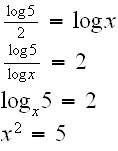# Simplifying logs

#### shocklightnin

1. The problem statement, all variables and given/known data
How do i solve for the subscript in:
(log (sub5)) / 2 = log(sub x)

2. Relevant equations

--

3. The attempt at a solution

the original question was:

(log(subx)7)(log(sub7)5)=2
solve for x.
however i dont get how to solve for a subscript....

#### icystrike

i don't get your question:

$$\frac{\log_{5}}{2}=\log_{x}$$

#### shocklightnin

i don't get your question:

$$\frac{\log_{5}}{2}=\log_{x}$$
thats the right equation, i was just wondering if anyone could help me solve for that 'x'?

#### icystrike#### Attachments

• 5.6 KB Views: 158

#### shocklightninthank you so much, you make understanding logs really easy! thanks.

#### icystrike

thank you so much, you make understanding logs really easy! thanks.
Its my pleasure (=

#### HallsofIvy

Warning, shocklightnin. Icystrike may have misunderstood your question and given a wrong answer!

I would interpret your question, since you specifically stated that "5" and "x" were "subscripts" (I would say "bases") as
"If
$$\frac{log_5(a)}{2}= log_x(a)$$
for some a, what is x?"

Then icystrike is answering a completely different question:
$$\frac{log(5)}{log(2)}= log(x)$$
which is, in a sense, the "reverse" of the original question!

If my interpretion is correct, since $log_x(a)= log(a)/log(x)$ and $log_5(a)= log(a)/log(5)$, where "log" on the right of each equation can be to any base, it follows that
$$\frac{log(a)}{log(5)}= 2\frac{log(a)}{log(x)}$$
Now the "log(a)" terms cancel out and we have

$$\frac{1}{log(5)}= \frac{2}{log(x)}$$

That is the equation you want to solve.

#### icystrike

Warning, shocklightnin. Icystrike may have misunderstood your question and given a wrong answer!

I would interpret your question, since you specifically stated that "5" and "x" were "subscripts" (I would say "bases") as
"If
$$\frac{log_5(a)}{2}= log_x(a)$$
for some a, what is x?"

Then icystrike is answering a completely different question:
$$\frac{log(5)}{log(2)}= log(x)$$
which is, in a sense, the "reverse" of the original question!

If my interpretion is correct, since $log_x(a)= log(a)/log(x)$ and $log_5(a)= log(a)/log(5)$, where "log" on the right of each equation can be to any base, it follows that
$$\frac{log(a)}{log(5)}= 2\frac{log(a)}{log(x)}$$
Now the "log(a)" terms cancel out and we have

$$\frac{1}{log(5)}= \frac{2}{log(x)}$$

That is the equation you want to solve.
there was a missing "a" to the equation , thus , i check with him if he was referring to the above equation that i mention. Hope he will reply (=

#### Borek

Mentor
the original question was:

(log(subx)7)(log(sub7)5)=2
Which seems to be

$$\log_x 7 \times \log_7 5 = 2$$

and as far as I can tell it was not yet mentioned...

### The Physics Forums Way

We Value Quality
• Topics based on mainstream science
• Proper English grammar and spelling
We Value Civility
• Positive and compassionate attitudes
• Patience while debating
We Value Productivity
• Disciplined to remain on-topic
• Recognition of own weaknesses
• Solo and co-op problem solving